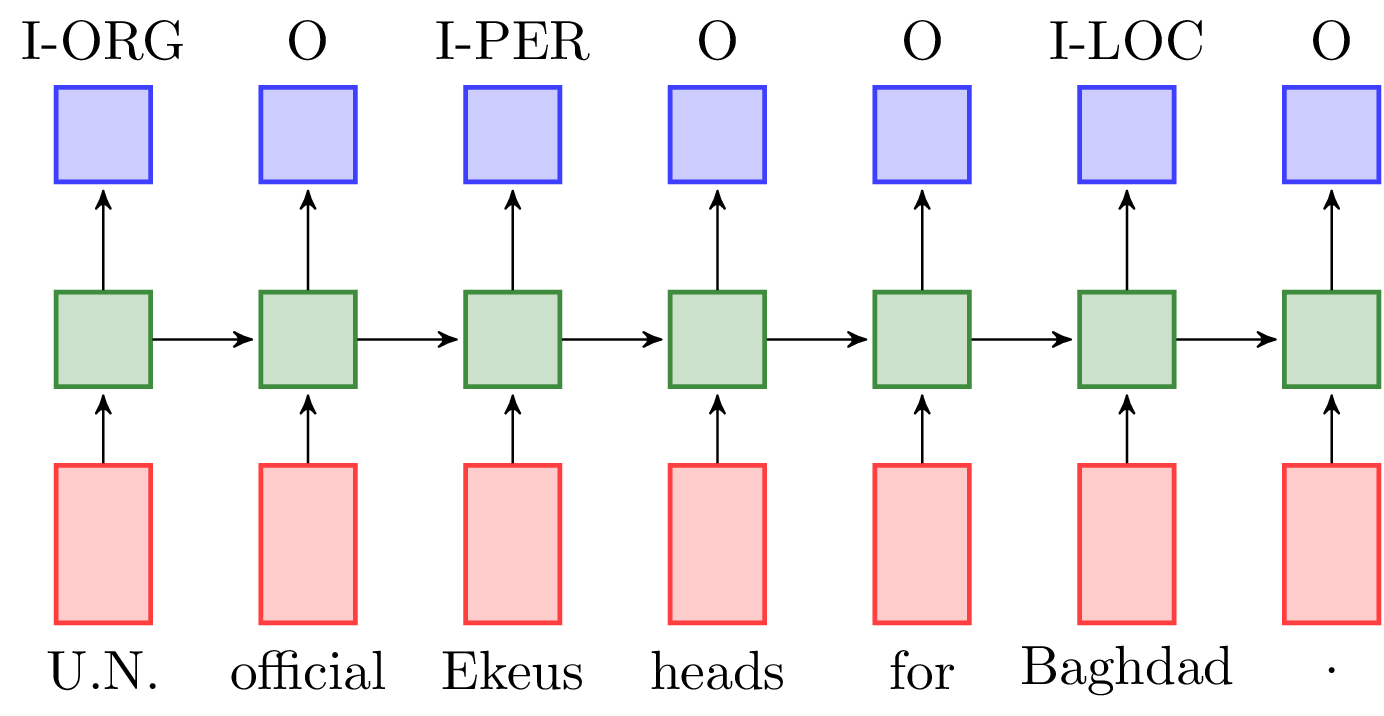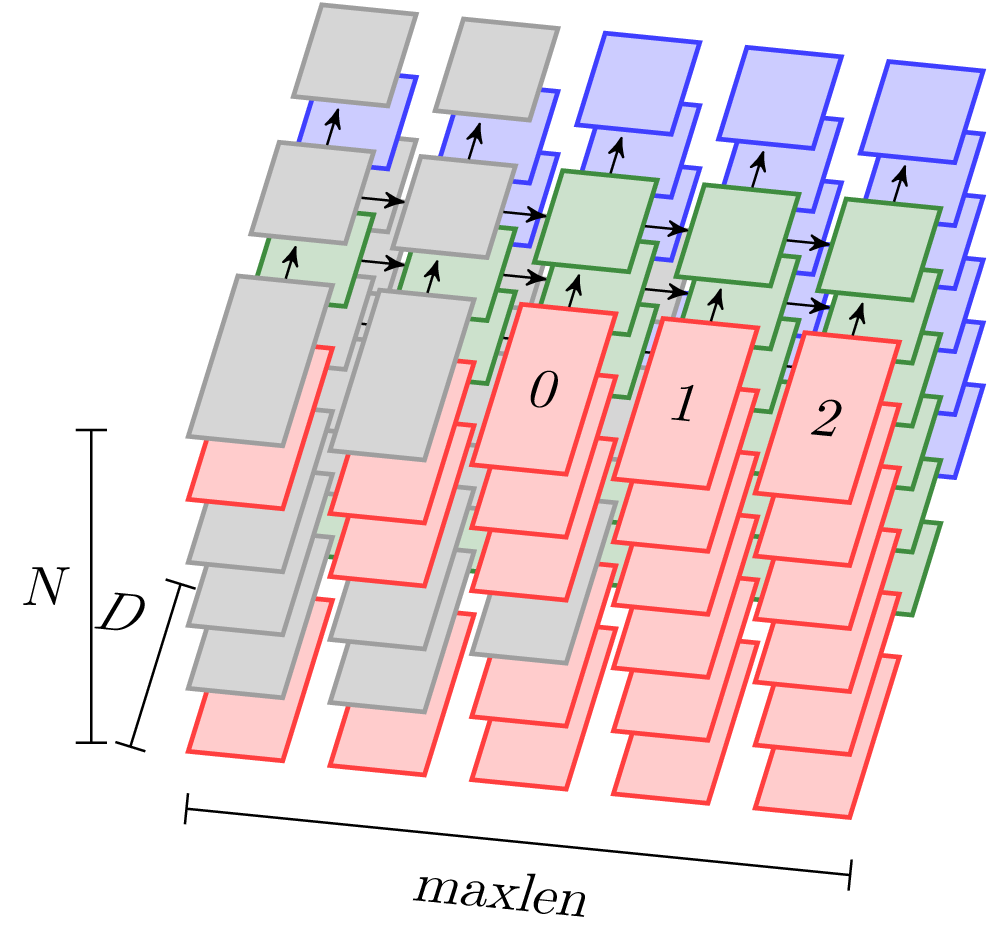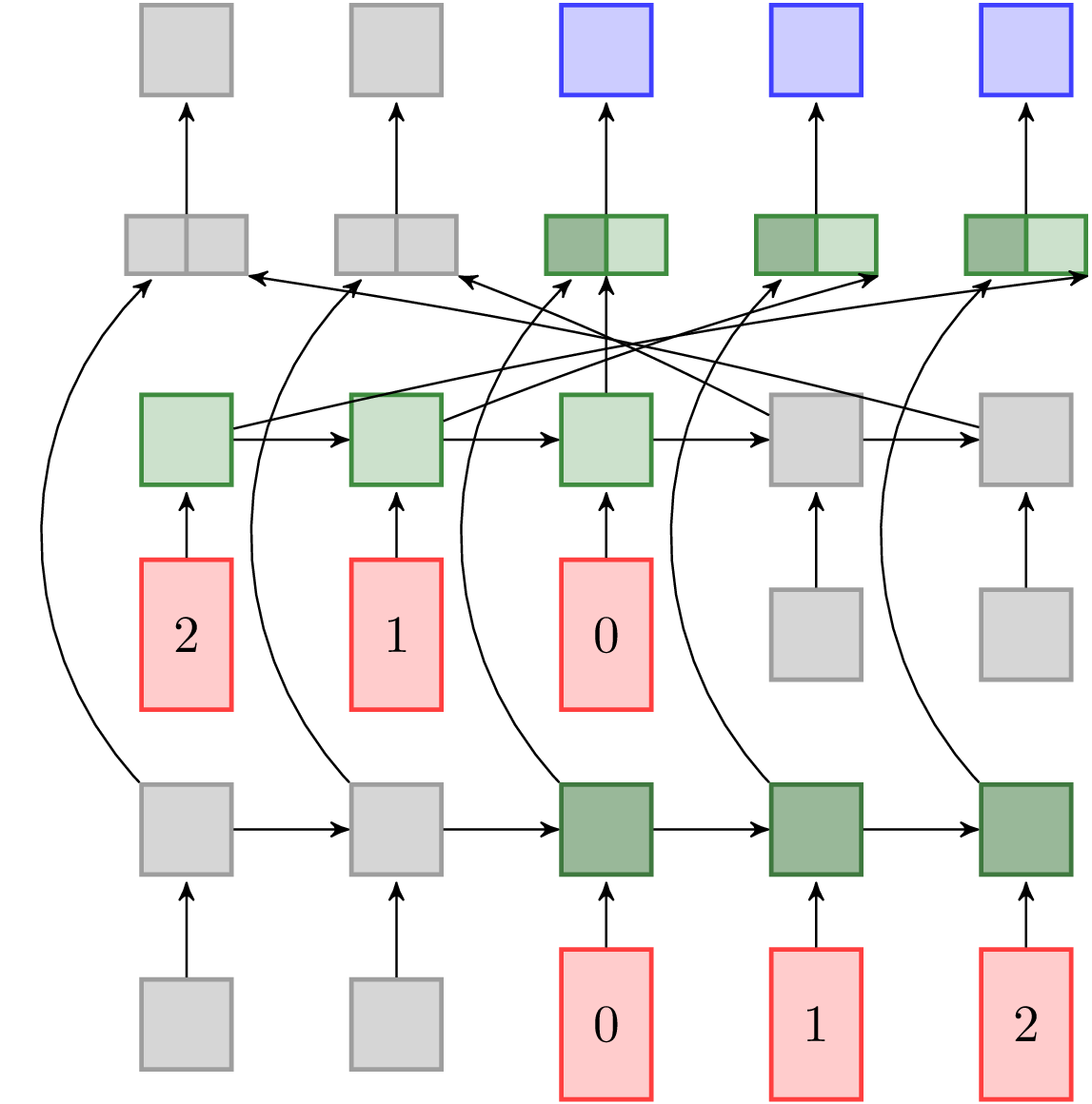# Masked bidirectional LSTMs with Keras

Bidirectional recurrent neural networks (BiRNNs) enable us to classify each element in a sequence while using information from that element’s past and future. Keras provides a high level interface to Theano and TensorFlow. In this post I’ll describe how to implement BiRNNs with Keras without using go_backwards (there are different ways to skin a cat).

## Sequence tagging

Sequence tagging falls in the many-to-many paradigm where there are as many labels as inputs.Examples of traditional NLP sequence tagging tasks include chunking and named entity recognition (example above).

## Sequence tagging with unidirectional LSTM

Although you can do a straight implementation of the diagram above (by feeding examples to the network one by one), you would immediately find that it is much to slow to be useful. To speed it up we need to vectorise the vectoriseable. This means that examples must be fed into the network in mini-batches.

The problem with this is of course that sequences are different lengths

• which is exactly why we are using RNNs in the first place—we want to be able to handle any length input.

There are a few ways to get variable length sequences and vectorisation. The one that seems to be the most popular is to fill in the sequences into an array block of size (N, maxlen, D), where maxlen is the length of the longest sequence in the set, and then zero-pad the rest. Then you enable masking on the sequence layer (LSTM/GRU/etc), which disables recurrent computation when the input is zero.Our model now looks like this (see this gist for the full preprocessing and training code):

max_features = len(word2ind)
embedding_size = 128
hidden_size = 32
out_size = len(label2ind) + 1

model = Sequential()
output_dim=embedding_size,
input_length=maxlen,



There are also subtleties when you want to break up long sequences into consecutive mini-batches (the RNN state must not be reset), but that is a story for another day (See Keras’ stateful RNNs).

## Looking both ways before crossing

With the model above we get to predict the current element’s label with information from the present and the past. But what if the next element (or the last one for that matter) helps to predict the current label?

For example if the current word is new then it is probably not a named entity, except if the next word is york.

Once again there are different ways to do this—you can do a pass through the sequence before labelling, or you can have RNNs going forward and backwards simultaneously as with a BiRNN.

There has been a bit of a debate about how to implement BiRNNs with Keras (See 1, 2, 3, 4, and 5), and to be honest I haven’t followed everything. It seems to me that there are two ways to think about it:

• Use a single input and then set the go_backwards flag (which is passed through to Theano/TF) on one of the passes. This implementation depends on how exactly masking works with go_backward (which still confuses me—maybe someone can explain to me to the current state of go_backward with masking?).
• The user must supply both normal and reversed inputs. The disadvantage here is that our input data size is doubled.

In my case I like to play with smaller datasets anyways so the second option looks much more understandable.## Reverse

The next crucial building block is a way to reverse sequences, and also their masks. One way to reverse sequences in Keras is with a Lambda layer that wraps x[:,::-1,:] on the input tensor. Unfortunately I couldn’t find a way in straight Keras that will also reverse the mask, but @braingineer created the perfect custom lambda layer that allows us to manipulate the mask with an arbitrary function.

Using the custom lambda:

from keras.backend import tf

# For theano back-end:
# return x[:,::-1,:]

# For tensorflow back-end:
return tf.reverse(x, [False, True, False])



(The tensorflow back-end doesn’t support [:, ::-1, :] giving NotImplementedError: Steps other than 1 are not currently supported, but luckily it works with .reverse().)

## Merge

Merge layers didn’t support masking until recently (See 1, 2), but works with Keras==1.0.6.

## Model

Using the good old Sequential setup our bidirectional RNN now looks like:

model_forward = Sequential()

model_backward = Sequential()
return tf.reverse(x, [False, True, False])

model = Sequential()



(Here is the gist of everything.)

Training is done by simply passing in the two versions of the input sequence to:

model.fit([X_train_f, X_train_b], y_train, batch_size=batch_size)


## Conclusion

Some other things that I want to explore:

• getting the hang of go_backwards`—it might simplify the model by making it ‘stackable’ (make it behave like a layer so we can stack a few together to create a deep BiRNN). It would probably need a lambda layer before the backwards RNN to align the mask. Come to think of it, we can make the current model stackable like that.
• use the functional API to share the embeddings between the forward and backward parts.

Next I’ll post the results of some experiments on the CoNLL named entity task.

Thanks for all @fchollet and the multitude of people working on Keras! (and Theano, and Tensorflow, and Python—opensource is amazing)

Written on April 2, 2016